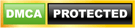# Between function in SQL Server

This function is used to select the values within a specified range. These values can be of Int type or Date data type or Text data type.

### Syntax:-

```SELECT column_name(s) FROM table_name WHERE column_name BETWEEN value1 AND value2```

In this article, we are going to use the following table for the examples:-

```CREATE TABLE [dbo]. [Employee]( [Empid] [Int] IDENTITY (1, 1) NOT NULL Primary key, [EmpNumber] [nvarchar](50) NOT NULL, [EmpFirstName] [nvarchar](150) NOT NULL, [EmpLAStName] [nvarchar](150) NULL, [EmpEmail] [nvarchar](150) NULL, [Salary] [int] NULL )```

We insert following data into the above tables:-

```Insert Into Employee (EmpNumber,EmpFirstName,EmpLAStName,EmpEmail,salary) Values('A001','Vivek','Johari','samir@abcmail.com',200000)```

```Insert Into Employee (EmpNumber,EmpFirstName,EmpLAStName,EmpEmail,salary) Values('A002','Amit','Kumar','amit@abcmail.com',100000)```

```Insert Into Employee (EmpNumber,EmpFirstName,EmpLAStName,EmpEmail,salary) Values('A003','Neha','Sharma','neha@abcmail.com',300000)```

```Insert Into Employee (EmpNumber,EmpFirstName,EmpLAStName,EmpEmail,salary) Values('A004','Chandra','Singh','vivek@abcmail.com',320000)```

```Insert Into Employee (EmpNumber,EmpFirstName,EmpLAStName,EmpEmail,salary) Values('A005','Avinash', 'Dubey','avinash@abcmail.com',250000)```

Example

For example, below query will give the Empnumber of all the employee whose salary lies between 250000 and 320000 (Including both the values (250000 & 320000 ))

```SELECT EmpNumber FROM employee WHERE salary BETWEEN 250000 AND 320000```

### Results

A003
A004
A005

Between command includes both the values (value1 and value 2) for returning the result. For example, suppose we want to get the records of the employees whose empid is lies between 1 and 4 then the query will be:-

`select  EmpNumber  from employee where empid between 1 and 4`

### Result

A001
A002
A003
A004

If we do not want to includes either of these specified minimum and maximum values or both these values , then we should use > or < operator instead of between command

For example below query will gives the EmpNumbers of employees whose empid are greater than 1 but less the 4

`select  EmpNumber  from employee where empid>1and empid`

A002
A003

## Not Between

If we want to select the values outside the specified range, we can use the NOT between function.

### Syntax:-

```SELECT column_name(s) FROM table_name WHERE column_name Not BETWEEN value1 AND value2```

For example, below query will give the Empnumber of all the employee whose salary lies outside the range specified by the minimum value of 250000 and maximum 320000.

```SELECT EmpNumber FROM employee WHERE salary NOT BETWEEN 250000 AND 320000```

### Result

A001
A002